Next: Normal Zeeman effect Up: Angular momentum and its Previous: Angular momentum and its

#### Angular momentum of coupled systems

We have seen earlier that we can represent the angular momentum states using the quantum numbers j and mj Now, suppose we have two sources of angular momentum represented by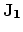and J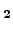. How may we represent the composite state?

It follows from the commutation relations for angular momentum that two representations are possible: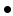Uncoupled representation: < j1mj1j2mj2 >Coupled representation: < j1j2jmj >

j1, j2, mj1, mj1 are the quantum numbers for the two angular momenta observables,and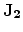.

j and mj are the corresponding quantum numbers of the composite angular momentum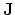where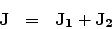The quantum number j is obtained from the so-called Clebsch-Gordan series: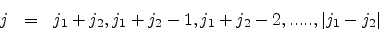and the quantum number mj is obtained as follows:

mj = - j to + j in integral steps. Similarly, mj1 = - j1 to + j1 in integral steps and mj2 = - j2 to + j2 in integral steps.

The two representations can be visualized pictorially using the vector-model of coupled angular momentum.

First we show the uncoupled representation below in the form of an animation.

Look carefully at the animation. The two blue arrows attached to the sides of the two cones represent the two angular momentum vectorsand. The red arrow represents the total angular momentum. ||, ||, the z components J1z and J2z respectively, all remain fixed. However || and its z component Jz do change. Hence in this representation, the corresponding quantum numbers j1, j2, mj1, mj1 may be specified together but not with j, mj.

Next we show the coupled representation below in the form of an animation.

Look carefully again at the animation. The two blue arrows attached to the sides of the two cones represent the two angular momentum vectorsandas before. So does the red arrow represent the total angular momentum. However note now that the two cones are now tilted to the vertical z-axis and haveas their common axis. ||, || remain fixed but the corresponding z components J1z and J2z vary. However || and the z component Jz remain fixed.andremain locked together, the angle between them remaining constant. Hence in this representation, the corresponding quantum numbers j1, j2, j, mj may be specified together but not with mj1 and mj2 . Also note that although mj1 and mj2 vary, their sum, mj remains fixed.

Quantization of angular momentum has many interesting fall outs as we shall see subsequently.Next: Normal Zeeman effect Up: Angular momentum and its Previous: Angular momentum and its
Abhijit Poddar
2007-09-27